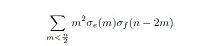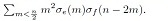##### Procedure for Exact Solutions of Nonlinear Pantograph Delay Differential Equations

Brahim Benhammouda, Hector Vazquez-Leal, Luis Hernandez-Martinez

Journal of Advances in Mathematics and Computer Science, Page 2738-2751
DOI: 10.9734/BJMCS/2014/11839

This work presents the application of the power series method (PSM) to find solutions of nonlinear delay differential equations of pantograph type (PDDEs). Three equations are solved to show that PSM can provide analytical solutions of PDDEs in convergent series form. The nonlinear pantograph cases study are: a first order equation, a second order equation, and a second order singular equation. Additionally, we present the post-treatment of the power series solutions with the Laplace-Pad´e (LP) resummation method as a powerful technique to find exact solutions. The proposed methodology possesses a simple procedure based on a few straightforward steps and it does not depend on a perturbation parameter.

##### Degree of Order Criteria of the Elements’ Deterministic Chains With Relations Between the Closest Neighbors

V. I. Ilyevsky

Journal of Advances in Mathematics and Computer Science, Page 2752-2764
DOI: 10.9734/BJMCS/2014/11490

For the first time ever, this work formulates definition of the degree of order for the non-random sequence of elements. Earlier, the only known parameter to evaluate a system’s degree of order was entropy. It has been shown that using entropy to evaluate the degree of order in deterministic chains of elements leads to a contradiction. Words built out of k different elements are being considered. Compositions are built from a random number of closed words that in total contain an equal quantity of each of the k elements. Hereby a new approach is being offered for the degree of order definition based upon comparison of the given composition with a high-symmetry word composition. Composition is described by means of the matrix of the number of pairs, in which the sum of elements in each line and each column are equal. Features of the pair matrix are studied. Pair matrices are expanded into the matrices of ideal states that describe compositions of words with shift symmetry. By means of this expansion the degree of order had been defined and its combinatory meaning had been revealed. A model of the ”cybernetic bug”, able to cut words in certain places, had been studied. It had been shown that repeated attacks by this cybernetic bug together with subsequent random patching of the cut words may, in a relatively short period of time, transfer composition to the ideal state.

##### (S, S´)-gap Shifts as a Generalization of Run-length-limited Codes

D. Ahmadi Dastjerdi, S. Jangjooye Shaldehi

Journal of Advances in Mathematics and Computer Science, Page 2765-2780
DOI: 10.9734/BJMCS/2014/11488

A generalization of Run-length-limited (RLL) codes has been introduced and its dynamical properties as a symbolic dynamical system under the shift map will be investigated. A formula for entropy and zeta function will be given and when our system is shift of finite type, its Bowen-Franks groups are obtained.

##### An Alternative Artificial Intelligence Technique for Detecting Outliers

Hegazy Zaher, Abd El-Fattah Kandil, Rehab Shehata

Journal of Advances in Mathematics and Computer Science, Page 2799-2810
DOI: 10.9734/BJMCS/2014/11194

Data are rarely perfect. Whether the problem is data entry errors or rare events. Outliers have two opposing properties. They can be noises that disturb regression and classification task. On the other hand, they can provide valuable information about rare phenomena, which can lead to knowledge discovery. This paper proposes a hybrid algorithm including K Nearest Neighbor and Support Vector Machine (KSVM) that detects outliers by taking the advantages of the two intelligent techniques, Support Vector Machine (SVM) and K Nearest Neighbour (KNN). Also a global efficiency measure introduced to compare different methods. Finally, a comparison between KNN, SVM, and KSVM is conducted using detection rate, accuracy rate, false alarm rate, true negative rate and the proposed global efficiency measure based on benchmark data called Milk data.

##### The Convolution Sums ∑m

Aeran Kim

Journal of Advances in Mathematics and Computer Science, Page 2811-2839
DOI: 10.9734/BJMCS/2014/10251

In this paper we obtain the convolution sum formulae offor n(∈) and an odd positive integer e and f. Moreover we obtain some identities induced from##### An Efficient Seven-point Hybrid Block Method for the Direct Solution of y''=f(x,y,y')

Journal of Advances in Mathematics and Computer Science, Page 2840-2852
DOI: 10.9734/BJMCS/2014/6749

A Self starting Stomer Cowell Hybrid Block Method of uniform Order 8 for Direct solution of General Second Order Initial Value Problems of Ordinary Differential Equations is presented in this paper. The method produces simultaneous approximation of the solution in a Block of Seven points at x=x(n+j) j=1,2,3,4,13/3,9/2 and 14/3 at once. The Block Analysis of this method shows that it is Zero stable and convergent. Numerical experiments show there superiority over the existing method.

##### About One Problem for the MAC Model for the Heat Conduction Equation

Olga Danilkina, Igor Neygebauer, Geminipeter Augustino Lyakurwa

Journal of Advances in Mathematics and Computer Science, Page 2853-2861
DOI: 10.9734/BJMCS/2014/11716

The aim of this work is to study a class of problems for continuum models in mechanics. We construct the MAC model for the parabolic equation which could include the physical solution appying Newton’s law of cooling. A notion of a generalized solution is introduced. We apply Galerkin method to prove the existence of a generalized solution. The proof of uniqueness of a generalized solution is based on the obtained energy inequality.

##### Effect of Retarding Force on Mass Flow Rates of Fluid at Different Temperatures Using Software Visualization Technique

Journal of Advances in Mathematics and Computer Science, Page 2715-2737
DOI: 10.9734/BJMCS/2014/10290

The designer of pipes normally faces the problems of how to design and prevent the pipes from leakage when used. To eradicate of reduces this problems, effects of retarding force on mass flow rate must take into consideration by compare the retarding force, mass flow rate of fluid at different temperature and with pipes of different radius. The fluid flow always takes place in a particular medium and pipe is one of the most common among the entire medium. During the flow process, retarding force has effect on the mass flow rates and always caused nagative effect by reduce the expected delivery product in term of mass flow rates. Main focus of this paper is to develop a mathematical model and software visualization to view the effect of retarding forces on the mass flow rate in term of visualization. Software Visualization (SV) is engaged in computer science by using computer graphics for communicating the structure and behavior of software or algorithms. It is mostly used for complex software. Application of this concept to real life complex situations has no boundary. Spillage of underground pipes has caused serious problems ranging from environmental pollution or degradation to economic shortage. It is caused by many factors either deliberate or unintended. C-sharp (C#) is the chosen program and this enable us to determine the mass flow rates patterns in relation to retarding force in form of graphical representation such as tables and graphics at different temperatures.

##### A Review of Wavelet-Based Image Processing Methods for Fingerprint Compression in Biometric Application

B. S. Emmanuel, M. B. Mu’azu, S. M. Sani, S. Garba

Journal of Advances in Mathematics and Computer Science, Page 2781-2798
DOI: 10.9734/BJMCS/2014/11944

A data compression algorithm is a signal processing technique used to convert data from a large format to one optimized for compactness. Huge volumes of fingerprint images that need to be transmitted over a network of biometric databases are an excellent example of why data compression is important. The cardinal goal of image compression is to obtain the best possible image quality at a reduced storage and transmission bandwidth costs. In this paper, a review of different methodological approaches to fingerprint image compression based on the wavelet algorithm is conducted. From the survey of the existing wavelet-based image compression methods, the problems that have been identified include: the limitation of WSQ standard to a compression ratio of 15:1 which could be improved with better algorithm. High complexity of image encoding process of the existing techniques is also a problem. Most of the existing methods require the generation of codebooks or lookup tables which require additional computational cost for implementation. Additionally, significant degradation in the biometric features of fingerprint at compression ratio higher than 15:1 remains a major challenge. Therefore, the investigation of an efficient compression method that can significantly reduce fingerprint image size while preserving its biometric properties (the core, ridge endings and bifurcations) is justified.